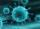Saving 9

An amount if \$ 2000 is invested at an interest of 5% per month. if \$ 200 is added at the beginning of each successive month but no withdrawals. Give an expression for the value accumulated after n months. After how many months will the amount have accumulated first exceed \$ 42000.

Result

n =  42

Solution:Leave us a comment of this math problem and its solution (i.e. if it is still somewhat unclear...):Be the first to comment!Next similar math problems:

1. Account operationsMy savings of php 90,000 in a bank earns 6% interest in a year. If i will deposit additional php 10,000 at the end of 6 months, how much money will be left if i withdraw php 25,000 after a year?
2. BankPaul put 10000 in the bank for 6 years. Calculate how much you will have in the bank if he not pick earned interest or change deposit conditions. The annual interest rate is 3.5%, and the tax on interest is 10%.
3. Six termsFind the first six terms of the sequence a1 = -3, an = 2 * an-1
4. Geometric progression 2There is geometric sequence with a1=5.7 and quotient q=-2.5. Calculate a17.
5. Theorem proveWe want to prove the sentence: If the natural number n is divisible by six, then n is divisible by three. From what assumption we started?
6. PopulationWhat is the population of the city with 3% annual growth, if in 10 years the city will have 60,000 residents?
7. Sequence - 5 membersWrite first five members of the sequence ?
8. LogarithmDetermine the number whose decimal logarithm is -3.8.
9. CardsSuppose that are three cards in the hats. One is red on both sides, one of which is black on both sides, and a third one side red and the second black. We are pulled out of a hat randomly one card and we see that one side of it is red. What is the probabi
10. GP membersThe geometric sequence has 10 members. The last two members are 2 and -1. Which member is -1/16?
11. SequenceFind the common ratio of the sequence -3, -1.5, -0.75, -0.375, -0.1875. Ratio write as decimal number rounded to tenth.
12. Five membersWrite first 5 members geometric sequence and determine whether it is increasing or decreasing: a1 = 3 q = -2
13. A perineumA perineum string is 10% shorter than its original string. The first string is 24, what is the 9th string or term?
14. Geometric progression 48,4√2,4,2√2
15. Geometric sequence 4It is given geometric sequence a3 = 7 and a12 = 3. Calculate s23 (= sum of the first 23 members of the sequence).
16. GP - 8 itemsDetermine the first eight members of a geometric progression if a9=512, q=2
17. VirusWe have a virus that lives one hour. Every half hour produce two child viruses. What will be the living population of the virus after 3.5 hours?